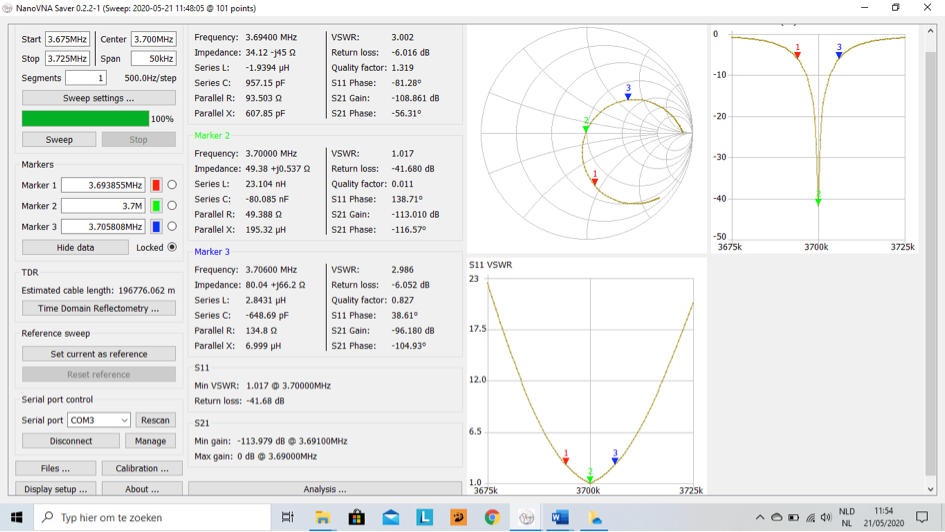top of page
Search
• xtgaby

# Magnetic loop 80 meter band.

Updated: Mar 14Calculated coil value of the loop is 6.4 uH as explained in de 40 meter band loop.

Target frequency = 3.700 KHz

Calculated value for the capacity = 290 pF.

I have used in this case RG58 with a capacitance of 100 pF / meter.

As you can see on this picture.

I had to change the small loop to insert the signal an ad 0,25 meter with the existing 1.30 meter for best SWR figures. Small loop becomes 1.55 meter.

Marker 1 is SWR = 3 lower frequency,

Marker 2 is SWR =1,

Marker 3 is SWR = 3 higher frequency.

This means that we have an SWR =3 bandwith of 3.706 – 3.694 = 12 KHz.SWR curve:Return loss curve: